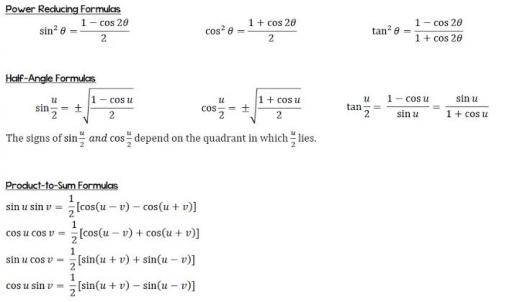# AP Calculus Quiz On Formulas!

10 Questions | Total Attempts: 140SettingsAdvanced Placement Calculus is a set of two distinct Advanced Placement calculus courses and exams offered by College Board. AP Calculus AB covers limits, derivatives, and integrals. AP Calculus BC covers all AP Calculus AB topics plus additional topics (including more integration techniques such as integration by parts, Taylor series, parametric equations, polar coordinate functions, and curve interpolations). This is a preparatory test ahead of the Calculus test.

• 1.
Transposing I = V/R for resistance R gives
• A.

I-V

• B.

V-I

• C.

I/V

• D.

VI

• 2.
When two resistors R1 and R2 are connected in parallel the formula 1/Rr = 1/R1 + 1/R2 the formula is used to determine the total resistance Rr. If R1 = 470 ohms, and R2 = 2.7k ohms, RT (in 3 significant figures) =
• A.

2.068 ohms

• B.

400 ohms

• C.

473 ohms

• D.

3170 ohms

• 3.
The formula for a focal length f of a convex lens is 1/f = 1/u + 1/v, where f = 4 and u = 6, v is
• A.

-2

• B.

1/12

• C.

12

• D.

-1/2

• 4.
Volume = mass/density, the density in (kg/m³) when mass is 2.532kg and volume is 162cm³ is:
• A.

0.01563kg/m3

• B.

410.2kg/m3

• C.

15630kg/m3

• D.

64.0kg/m3

• 5.
PV = mRT is the characteristic gas equation. When P = 100 × 10³, V = 4.0, R = 288, and T = 300, what is the value of m?
• A.

4.630

• B.

313600

• C.

0.216

• D.

100592

• 6.
The volume V2 of a material when temperature is increased is by V2 = V1 [1 + y (t2 – t1)]. The value of t2 when V2 = 61.5cm³, V1 = 60cm³, y = 54 × 10^-6 and t1 = 250 is
• A.

213

• B.

463

• C.

713

• D.

28028

• 7.
The current i amperes flowing in a capacitor at time t seconds is given by i = 10(1 − e^−t/CR), where resistance R is 25 × 10³ ohms and capacitance C is 16 × 10^−6 farads. When current i reaches 7 amperes, the time t is:
• A.

-0.48s

• B.

0.14s

• C.

0.21s

• D.

0.48s

• 8.
A formula used for calculating the resistance of a cable is R = ρl/a . A cable’s resistance R = 0.50 ohms, its length l is 5000 m and its cross-sectional area a is 4 × 10-⁴ m². The resistivity ρ of the material is:
• A.

6.25 x 107 ohms m

• B.

4 x 10-8 ohms m

• C.

2.5 x 107 ohms m

• D.

3.2 x 10-7 ohms m

• 9.
Current I in an electrical circuit is given by I = E − e/ R + r. Transposing for R gives:
• A.

(E - e - Ir)/I

• B.

(E - e)/(I + r)

• C.

(E - e)(I + r)

• D.

(E - e)/Ir

• 10.
Transposing t = 2π √l/g for g gives:
• A.

(t − 2π)² / l

• B.

(2π/ t) l²

• C.

(√t/2π) / l

• D.

4π²l / t²

Related TopicsBack to top